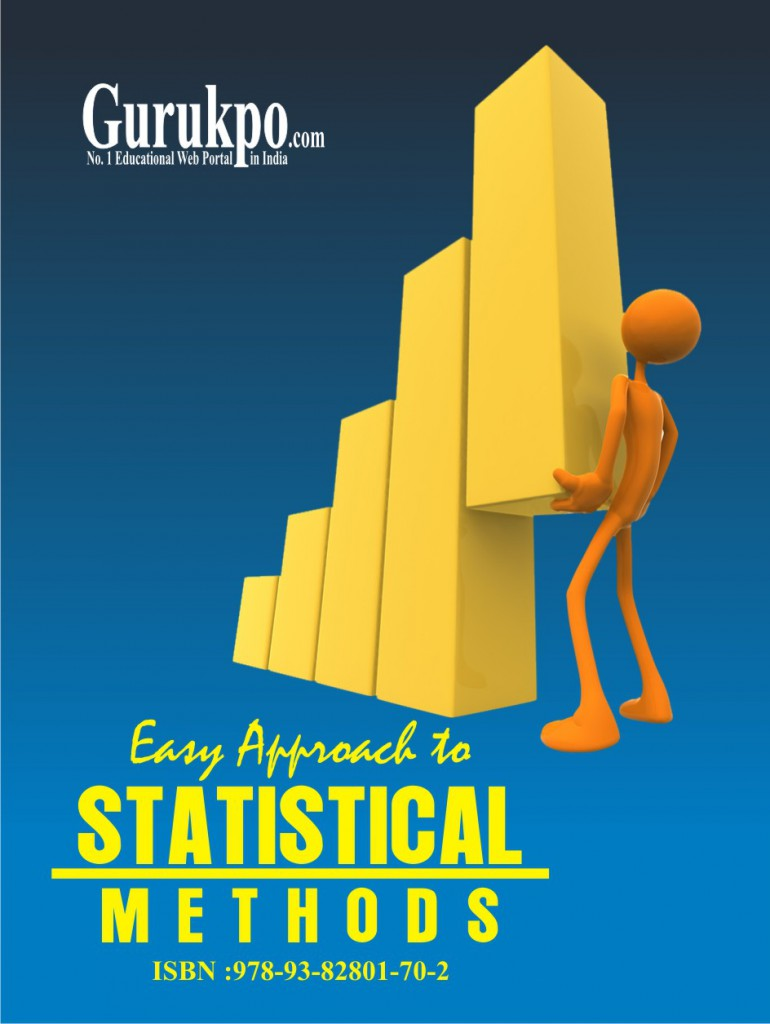# Statistical Methods

Statistics: An Introduction,Classification,Tabulations,Measure of Central Tendency,Measure of Dispersion,Probability,Probability Distribution,Theory of Estimation,Sampling Theory,Tests of significance – ‘t’ test & ‘z’ test,Chi-Square Test ( X2 Test),Analysis of Variance,Decision Theory,Correlation,Regression Analysis,Time Series Analysis,Business Forecasting,Skewnness,Moments and Kurtosis,Case Study,Key Terms